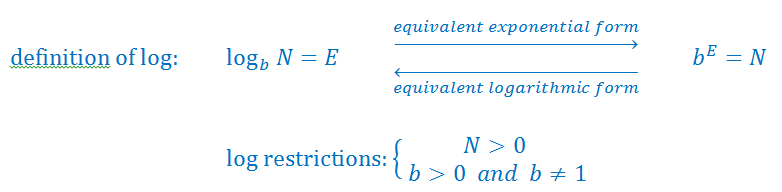# Converting from logarithmic form to exponential form

#### You’re one step closer to a better grade.

Learn with less effort by getting unlimited access, progress tracking and more.Learn More
0/1

### Introduction

#### Lessons

1.0/10

### Examples

#### Lessons

1. Rewriting the Equations in Exponential Form
Convert from log form to exponential form
1. $\log_28 = 3$
2. 123 = 4$\log_b5$
2. Solving Equations by Converting From Logarithmic to Exponential Form
Solve for x
1. $\log_3x = 4$
2. $\log_525 = x$
3. given: $\log_9w = 0.5$ and $\log_2x = w$

3. Solve for ${y}$:
$\log_5( \frac{y}{2}) = x$
1. A Logarithmic Expression Inside Another Logarithmic Expression
Solve for ${x}$:
$\log_8( \log_7(5-x)) = \frac{1}{3}$
1. Extension: Solving Equations by Converting From Logarithmic to Exponential Form
1. solve for ${ y: }$
$\log_{10}0.00001 = y$
2. solve for ${ x: }$
3 = 5$\log_x8$
3. solve for ${ y: }$
$\log_3(5y-30)=2x+20\$

## Become a Member to Get More!

• #### Easily See Your ProgressWe track the progress you've made on a topic so you know what you've done. From the course view you can easily see what topics have what and the progress you've made on them. Fill the rings to completely master that section or mouse over the icon to see more details.

• #### Make Use of Our Learning Aids###### Practice Accuracy

See how well your practice sessions are going over time.

Stay on track with our daily recommendations.

• #### Earn Achievements as You LearnMake the most of your time as you use StudyPug to help you achieve your goals. Earn fun little badges the more you watch, practice, and use our service.

• #### Create and Customize Your AvatarPlay with our fun little avatar builder to create and customize your own avatar on StudyPug. Choose your face, eye colour, hair colour and style, and background. Unlock more options the more you use StudyPug.

## What is exponential form in math?

The log form and exponential form are actually inverses of each other. Exponentials happen when a number is raised to a certain power. It is a shorter way to show that a number is repeatedly multiplied a number of times by itself. An example of an exponential form number would be that in order to show 3x3x3x3, we'd instead write 34. The exponent is the little number 4, and it shows how many times the number is to be multiplied by itself. The base is 3, and it is what is being multiplied.

A logarithm of a number, on the other hand, is the exponent that a base has to be raised to make that number.

You probably recall how to change exponential form to radical form. But in this chapter, we're looking at something a little different. How do we convert log form to exponential form and vice versa? What's does converting log vs exponential form ultimately look like? Let's explore this through an example.

Question: Convert $lo{g_2}\;8\; = 3$into exponential form.

Solution:

$lo{g_2}\;8\; = 3$

Convert into this form: ${b^E} = N$

${2^3} = 8$
How does this work? Let's take a step-by-step look into how we change the form of the equation. The relationship between log and exponential form makes converting between the two easy. ${b^E} = N$ is equalled to $lo{g_b}\left( y \right)\; = x$ is the relationship that we're referring to. What exactly does the letters in ${b^E} = N$mean?
Using the relationship, we can easily see how by looking at a log form equation, we can convert it to exponential form. Simply by moving the corresponding parts of the log form equations into ${b^E} = N$ format, you can find the exponential form of log. To recap: In order to change a logarithmic form function to an exponential one, first find the base, which is the little number next to the word "log". The base doesn't change sides, but the other numbers (the exponent and the "number") do. Lastly, remove the word "log" from the equation and you'll have successfully converted the equation!